# Chemical Kinetics Class 12 Important Questions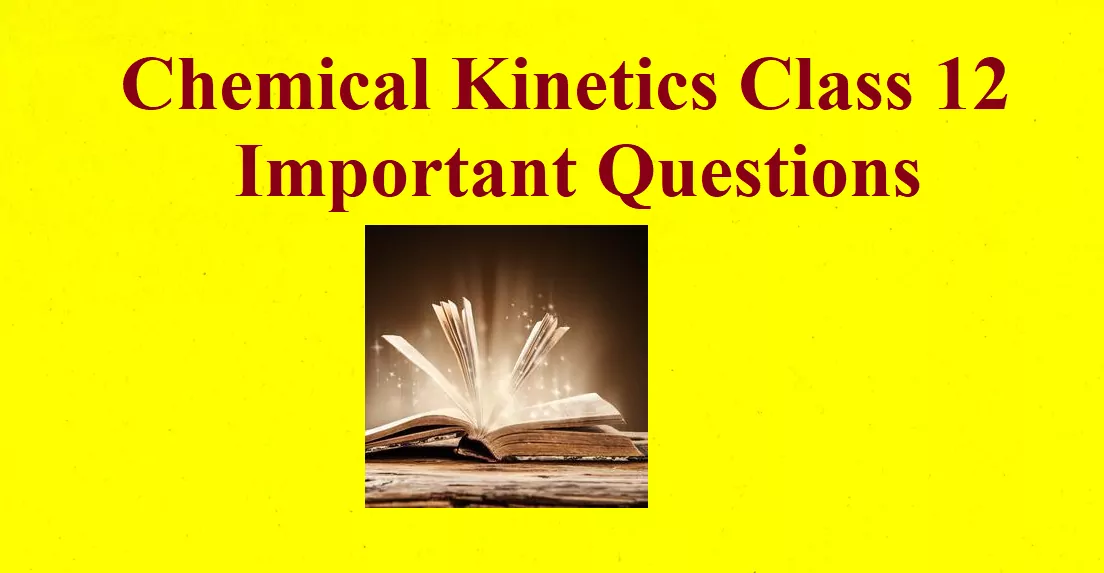Chemical Kinetics Class 12 Important Questions

Que 1. Define the following:
(a) Rate of reaction
(b) Specific reaction rate or rate constant.
Ans 1. (a) The change in concentration of any reactant or product per unit time is called the rate of reaction.
(b) It is equal to the rate of reaction when the molecular concentration of reactant is at unity.

Que 2. The rate law for a reaction is Rate = K [A] [B]5/2. Can the reaction be an elementary process? Explain
Ans 2. No, because an elementary reaction is a chemical reaction in which one or more chemical species react directly to form products in a single reaction step.

Que 3. How does catalyst alter the rate of a reaction?
Ans 3. It increases the rate of reaction by providing a new path having low activation energy.

Que 4. Explain the following X Y
(a) The conversion of molecules X to Y follows the second-order kinetics. If the concentration of X is decreased 3 times, how will it affect the rate of formation of Y?
(b) If a reaction has s-1 as the unit of the rate constant. What will be the order of the reaction?
Ans 4. (a) Rate = k[A]2 = k[1/3A]2 = 1/9k[A]2
The rate of formation will become 1/9 times.
(b) First-order reaction

Chemical Kinetics Class 12 Important Questions

Que 5. Identify the order of a reaction from the following rate constant:
k = 2.3 × 10−5 L mol-1 s-1
Ans 5. Second-order

Que 6. For the reaction R → P, the concentration of a reactant changes from 0.03 M to 0.02 M in 25 min. Calculate the average rate of reaction using units of time both in minutes and seconds.
Ans 6.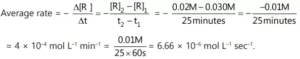Que 7. Calculate the overall order of a reaction that has the rate expression.
Rate = k [A]1/2[B]3/2.
Ans 7. Order of reaction =  ½ + 3/2 = 2  i.e. Second order reaction.

Que 8. What value of k is predicted for the rate constant by the Arrhenius equation is T → ∞? Is this value physically reasonable?
Ans 8. From the equation k = Ae-Ea/RT. If T → ∞ k → A so that Ea = 0. This is not feasible.

Chemical Kinetics Class 12 Important Questions

Que 9. During the analysis of the products, even when a portion of the mixture is removed, the rate of reaction in the remaining mixture is not affected. Explain.
Ans 9. The rate of reaction depends on concentration, which remains constant even if a portion of the mixture is removed, i.e. amount of substance is independent of the concentration and hence remains unaffected.

Que 10. Define the term activation energy.
Ans 10. The additional energy which is required by the molecules of reactants to cause an effective collision is called activation energy.

Que 11. Write the difference between order and Molecularity of reaction.
Ans 11.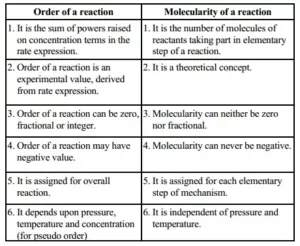Que 12. Why can’t the molecularity of any reaction be equal to zero?
Ans 12. The Molecularity of a reaction means the number of molecules of the reactants taking place in an elementary reaction. Since at least one molecule must be present, so that molecularity will be at least one.

Que 13. For the reaction A + B → C + D, the rate law equation = K1 [A] + K2 [A] [B], where K1 and K2 represent two different constants and the products are formed by two different mechanisms. Explain the relative magnitudes of the rates of two individual mechanisms?
Ans 13. The rate of formation of products for both the reaction mechanisms must be of the same order of magnitude. Different magnitude reflects only the rate of reaction of the one which is faster among the two mechanisms. Also, it involves the rate of formation of products from A as well as products from A and B.

Que 14. Give one example of a reaction where order and molecularity are equal?
Ans 14. 2HI → H2 + I2 (Order = Molecularity = 2)

Que 15. The decomposition of the following reaction follows first-order kinetics
3 A(g) → 2 B(g) + 2 C(g) At the beginning of the reaction, only A is present. The pressure developed after 10 min. and infinite time is 3.5 and 4 atm respectively. Calculate t75.
Ans 15.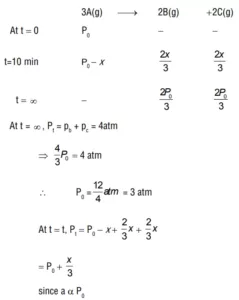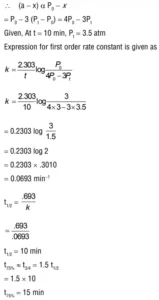Chemical Kinetics Class 12 Important Questions

Que 16. The rate constant for a first-order reaction is 60 s–1. How much time will it take to reduce the initial concentration of the reactant to its 1/16th value?
Ans 16.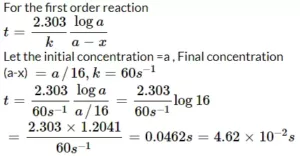Que 17. What will be the effect of temperature on the rate constant?
Ans 17. The rate constant of a reaction is nearly doubled with rising in temperature by 10°C. The dependence of the rate constant on temperature is given by the Arrhenius equation, k = Ae− E a/RT, where A is called frequency factor and Ea is the activation energy of the reaction.

Que 18. i) What is the unit for K for the following reaction rate law:
(a) Rate = K[A][B]0
(b) Rate = K[A]0[B]0
(ii) Why is the use of the instantaneous rate of reaction preferred over the average rate of reaction?
Ans 18. (i) (a) s-1
(b) moleL-1s-1
(ii) The rate of reaction continuously decreases with time (except for zero-order reaction) and Therefore, the average rate has no significance for the reaction.

Que 19. For a reaction R → P, the rate becomes 2 times when the concentration of reactant A is increased 4 times. What is the order of reaction?
Ans 19. r = k(a)n ⇒ 2r = k(4a)n ⇒ 2 = 4n ⇒ n = 0.5

Que 20. Show that in a first order reaction, time required for completion of 99.9% is 10 times of half life (t1/2) of the reaction.
Ans 20. When reaction is completed 99.9%, [R]n = [R]0 – 0.999[R]0
k = 2.303/t (log R0/R) = 2.303/t (logR0/[R]0 – 0.999 R0)
= 2.303/t log103
t = 6.909/k
For half‐life of the reaction t1/2 = 0.693/k
t/t1/2 =6.909/0.693 =10
t = 10 t1/2

Chemical Kinetics Class 12 Important Questions

Que 21. (a) The half‐life for the radioactive decay of 14C is 5730 years. An archaeological artifact containing wood had only 80% of the 14C found in a living tree. Estimate the age of the sample.
(b) A reaction is first order in A and second order in B.
(i) Write the differential rate equation.
(ii) How is the rate affected on increasing the concentration of B three times?
(iii) How is the rate affected when the concentrations of both A and B are doubled?
Ans 21. (a) Given t1/2= 5730 years, [R]o = 100, [R] = 80
K=0.693/t
K = 0.693/5730 = 1.21 × 10–4 year–1
We know
t =2.303/K (log[R]o/[R])
=2.303/1.21 × 10 log100/80 = 1.9033 × 10-4 (log100 – log80)
= 1.9033 × 10–4 (2 ‐ 1.9031) = 1.9033 × 10–4 (0.0969) = 1845 years
(b) (i) Rate = K[A]1 [B]2
(ii) Rate = K[A]1 [3B]2 = 9K[A]1 [B]2 hence, it becomes 9 times.
(iii)Rate = K[2A]1 2[B]2 = 8K[A]1 [B]2 hence, it becomes 8 times

Que 22. (a) Why the rate of reaction does not remain constant throughout?
(b) What is the half-life period of a reaction?
(c) The rate of decomposition of a substance A becomes 8 times when its concentration is doubled. What is the order of the reaction?
Ans 22. (a) The rate of a reaction depends on the concentration of the reactant and the concentration of reactant decreases with the passage of time.
(b). It is the time required by the reaction to undergo 50% completion or the time by which the concentration of the reactant reduces to half of its initial value.
(c). Rate = k[A]n => r = k[A]n ………(i)
8r = k[2A]n ……………..(ii)
dividing (ii) by (i)
8r/r = k[2A]n /k[A]n  => 8 = n => n = 3,
Order is 3

Que 23. If the decomposition of nitrogen oxide as 2N2O5→ 4NO2 + O2 follows the first-order kinetics.
(i). Calculate the rate constant for a 0.05 M solution if the instantaneous rate is 1.5 x 10-6 molL-1s-1?
ii) What concentration of N2O5 would give a rate of 2.45 x 10-5moll-1s-1?

Ans 23. (i) We know that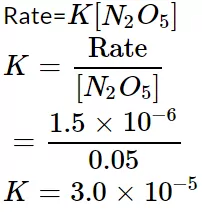(ii). Rate = K[N2O5] [N2O5] = Rate/k = 2.45X10-5/3.0X10-5
= 0.82

Que 24. (a) Determine the units of the rate constant for the first and zero-order reactions.
(b) Show that the time required for the completion of 99% of the first-order reaction is twice the 90% of completion of the reaction.
Ans 24. (a). K = (mol)1 – n Ln – 1 S–1
For zero order, n = 0
So, K = (mol)1 – 0 L0 – 1 S–1 = S–1 mol L–1
For first order, n = 1
K = (mol)1 – n Ln – 1 S–1
So, K = (mol)1 – 1 L1 – 1 S–1 = S–1

(b) For a first-order reaction,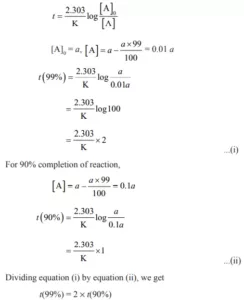Chemical Kinetics Class 12 Important Questions